# Exam-Style Questions on Vectors

## Problems on Vectors adapted from questions set in previous GCSE Extended and Higher exams.

### 1.

IGCSE Extended

OABC is a parallelogram with O as origin. The position vector of A is $$a$$ and the position vector of C is $$c$$.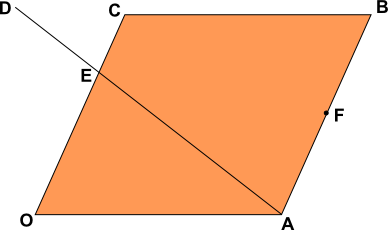F is the mid-point of AB and the point E divides the line OC such that OE:EC = 2:1.

The point E also divides the line AD such that AE:ED = 3:2.

Find the following in terms of $$a$$ and $$c$$.

(a) $$\overrightarrow{OB}$$

(b) $$\overrightarrow{AC}$$

(c) $$\overrightarrow{AE}$$

(d) the position vector of F.

(e) $$\overrightarrow{AD}$$

(f) $$\overrightarrow{BD}$$

### 2.

GCSE Higher

(a) Shape $$A$$ is translated to shape $$B$$ using the vector $$\begin{pmatrix}m\\n\\ \end{pmatrix}$$. What are the values of $$m$$ and $$n$$?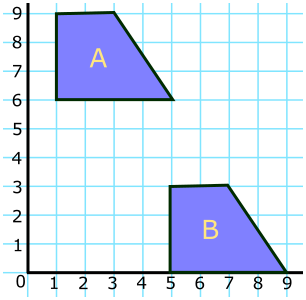(b) Vectors $$a, b, c, d$$ and $$e$$ are drawn on an isometric grid. Write each of the vectors $$c, d$$ and $$e$$ in terms of $$a$$ and/or $$b$$.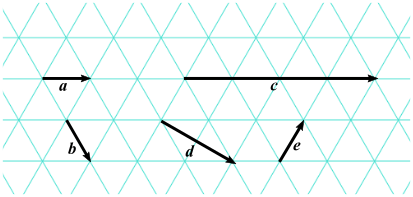### 3.

GCSE Higher

ABCD is a quadrilateral. The points E, F, G and H are the midpoints of the sides of this quadrilateral.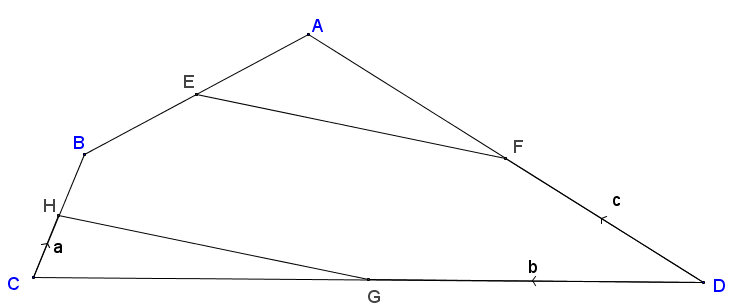$$\vec{CH} = a, \vec{DG} = b \text{ and } \vec{DF} = c$$

Show that HG is parallel to EF.

### 4.

IGCSE Extended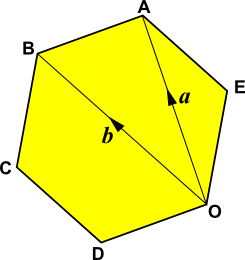ABCDOE is a regular hexagon with O as origin. The position vector of A is $$a$$ and the position vector of B is $$b$$.

Find the following in terms of $$a$$ and $$b$$.

(a) $$\overrightarrow{BA}$$

(b) $$\overrightarrow{OE}$$

(c) the position vector of C.

If the sides of the hexagon are each of length 10cm calculate:

(d) the size of angle $$BCD$$.

(e) the area of triangle $$BCD$$.

(f) the length of the line from B to D.

(g) the area of the hexagon.

### 5.

GCSE Higher

Consider a triangle ABC where M is the midpoint of AB and F is the point on BC where BF:FC = 3:4.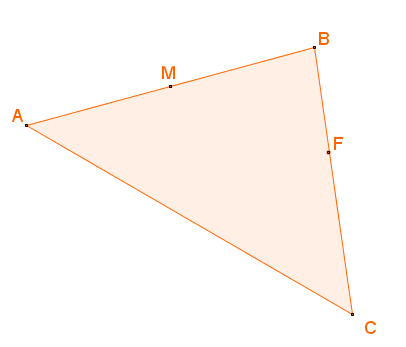(a) If $$\overrightarrow{AB}=b$$ and $$\overrightarrow{AC}=c$$ work out $$\overrightarrow{MF}$$ in terms of $$b$$ and $$c$$ giving your answer in its simplest form.

(b) Use your answer to part (a) to explain whether MF is parallel to AC or not.

### 6.

GCSE Higher

In the parallelogram OABC two of the sides can be represented by vectors $$a$$ and $$c$$.

$$\overrightarrow{OA} = a$$ and $$\overrightarrow{OC} = c$$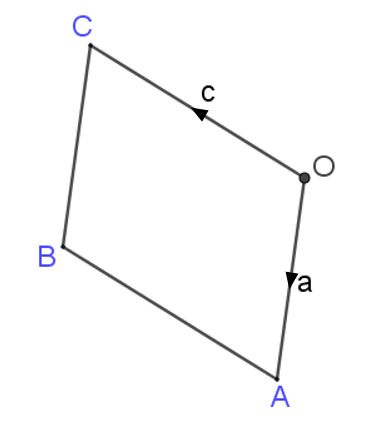$$X$$ is the midpoint of the line $$AC$$.

$$OCD$$ is a straight line such that $$OC:CD = k:1$$

Given that $$\overrightarrow{XD} = 3c - \frac12 a$$ find the value of $$k$$.

### 7.

IGCSE Extended

(a) If A is the point (3,5) write down the position vector of A.

(b) If B is the point (6,9) find $$\mid\overrightarrow{AB} \mid$$ the magnitude of $$\overrightarrow{AB}$$.

The following diagram is not to scale.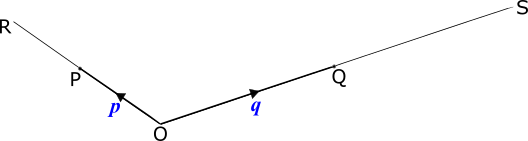$$O$$ is the origin, $$\overrightarrow{OP}=p$$ and = $$\overrightarrow{OQ}=q$$.

$$OP$$ is extended to $$R$$ so that $$OP=PR$$.

$$OQ$$ is extended to $$S$$ so that $$OQ=QS$$.

(c) Write down $$\overrightarrow{RQ}$$ in terms of $$p$$ and $$q$$.

(d) $$PS$$ and $$RQ$$ intersect at $$M$$ and $$RM=2 MQ$$.

Use vectors to find the ratio $$PM:PS$$, showing all your working.

### 8.

GCSE Higher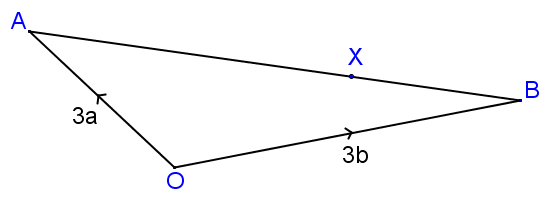In the diagram above (not drawn to scale) $$X$$ is the point on $$AB$$ such that $$AX:XB = 9:4$$.

The position vector of $$A$$ is $$3a$$ and the position vector of $$B$$ is $$3b$$.

Find the value of $$k$$ if $$\vec{OX} = k(4a + 9b)$$ where $$k$$ is a scalar quantity.

The exam-style questions appearing on this site are based on those set in previous examinations (or sample assessment papers for future examinations) by the major examination boards. The wording, diagrams and figures used in these questions have been changed from the originals so that students can have fresh, relevant problem solving practice even if they have previously worked through the related exam paper.

The solutions to the questions on this website are only available to those who have a Transum Subscription.

Exam-Style Questions Main Page

Search for exam-style questions containing a particular word or phrase:

To search the entire Transum website use the search box in the grey area below.

Kausar Begum Khan, Kenya

Friday, January 26, 2018

"This is a fabulous website! I cannot thank you enough."

Tuesday, November 6, 2018

"Transum is so resourceful. The resources are so child-friendly that my students love its usage.It has a wide range of topics and caters to most of my needs. 'Thankyou" will be too small a word to express my gratitude to the content developers of this site."

Ahmed Jaja, Ghana

Thursday, November 22, 2018

"It was very useful for me.I recommend it to every math student.Thank you."

Tuesday, February 5, 2019

"A very great site.Nice questions."

Yuli, Woodmansterne

Tuesday, April 2, 2019

"This website is amazing it helps me with my maths to get good marks in my upcoming test. Thanks."

Do you have any comments? It is always useful to receive feedback and helps make this free resource even more useful for those learning Mathematics anywhere in the world. Click here to enter your comments.For All: